Related Articles

# Regularization in Machine Learning

• Last Updated : 29 Jun, 2021

Overfitting is a phenomenon that occurs when a Machine Learning model is constraint to training set and not able to perform well on unseen data.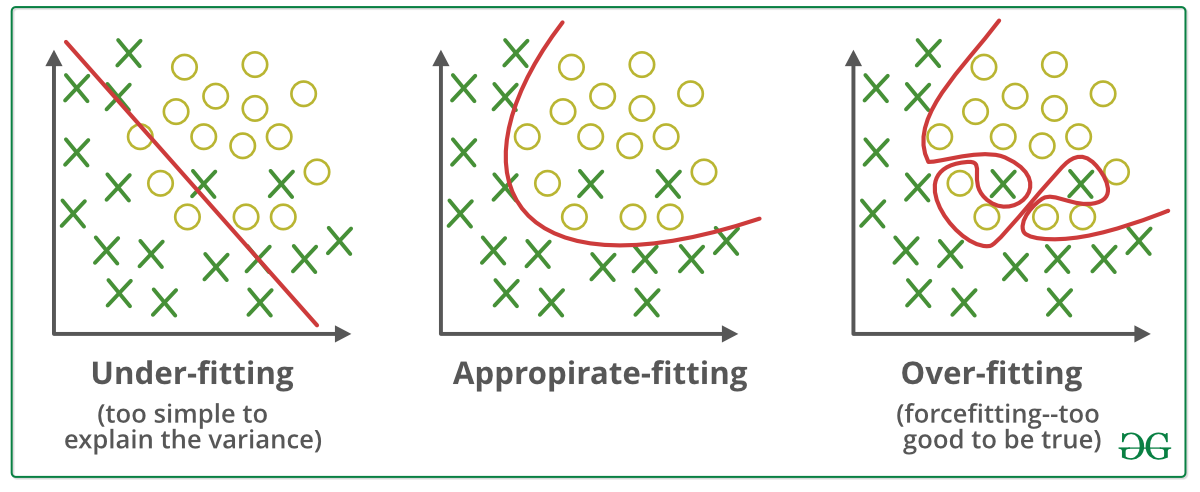Regularization is a technique used to reduce the errors by fitting the function appropriately on the given training set and avoid overfitting.
The commonly used regularization techniques are :

1. L1 regularization
2. L2 regularization
3. Dropout regularization

A regression model which uses L1 Regularization technique is called LASSO(Least Absolute Shrinkage and Selection Operator) regression.
A regression model that uses L2 regularization technique is called Ridge regression
Lasso Regression adds “absolute value of magnitude” of coefficient as penalty term to the loss function(L).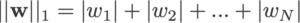Ridge regression adds “squared magnitude” of coefficient as penalty term to the loss function(L).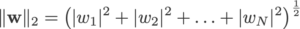NOTE that during Regularization the output function(y_hat) does not change. The change is only in the loss function.

The output function: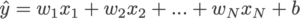The loss function before regularization: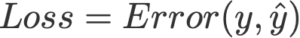The loss function after regularization: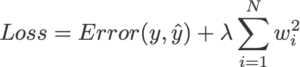We define Loss function in Logistic Regression as :

```                    L(y_hat,y) = y log y_hat + (1 - y)log(1 - y_hat)

```

Loss function with no regularization :

`                L = y log (wx + b) + (1 - y)log(1 - (wx + b))   `

Lets say the data overfits the above function.

Loss function with L1 regularization :

`                      L = y log (wx + b) + (1 - y)log(1 - (wx + b)) + lambda*||w||1    `

Loss function with L2 regularization :

`                      L = y log (wx + b) + (1 - y)log(1 - (wx + b)) + lambda*||w||22    `

lambda is a Hyperparameter Known as regularization constant and it is greater than zero.

`                           lambda > 0`

Attention reader! Don’t stop learning now. Get hold of all the important Machine Learning Concepts with the Machine Learning Foundation Course at a student-friendly price and become industry ready.

My Personal Notes arrow_drop_up